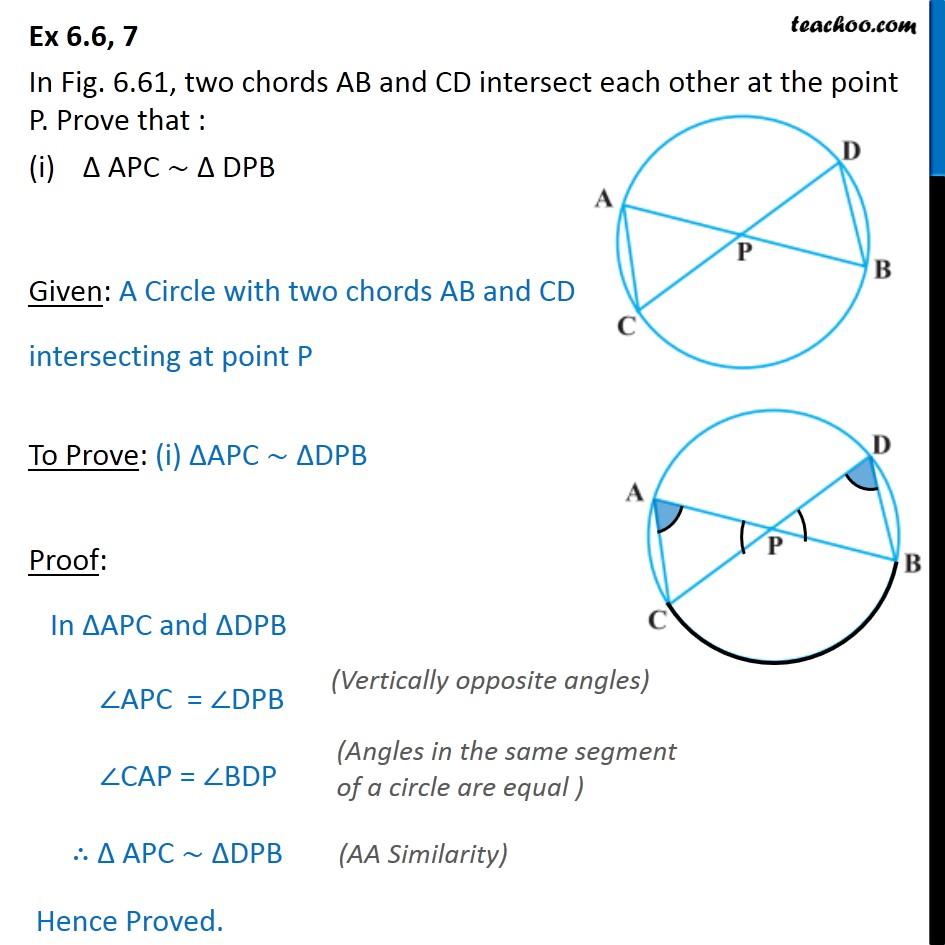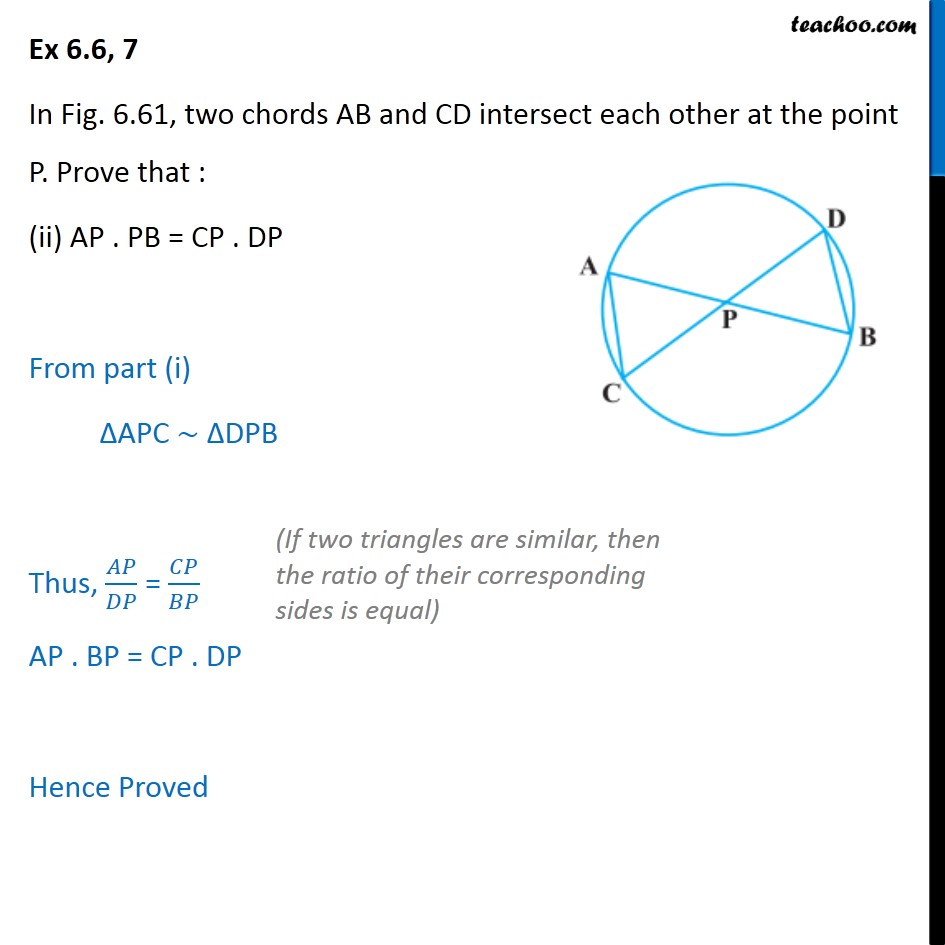Important questions of Triangle (in Geometry)

Chapter 6 Class 10 Triangles
Serial order wiseLearn in your speed, with individual attention - Teachoo Maths 1-on-1 Class

### Transcript

Question 7 In Fig. 6.61, two chords AB and CD intersect each other at the point P. Prove that : APC DPB Given: A Circle with two chords AB and CD intersecting at point P To Prove: (i) APC DPB Proof: In APC and DPB APC = DPB CAP = BDP APC DPB Hence Proved. Question 7 In Fig. 6.61, two chords AB and CD intersect each other at the point P. Prove that : (ii) AP . PB = CP . DP From part (i) APC DPB Thus, / = / AP . BP = CP . DP Hence Proved